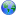Last update August 5, 2009

# lvalue

An expression that represents an object or a function and that can be both examined and altered. Contrast with rvalue.source

Typically an lvalue is a variable, returned reference, pointer being dereferenced, indexed array element, or class instance (Object).

Modifying operations like ++, --, +=, *=, =, member function calls, and such may be applied to lvalues.

The dereference operator *expr turns rvalues into lvalues. The reference or address-of operator &expr turns lvalues into rvalues.

Examples:```// D2 code. class C { int a; this() {} } struct S { int a; } int foo; int bar() { return foo; } int* baz() { return &foo; } ref int qux() { return foo; } C getC() { return new C(); } S s; S getS() { return s; } void main() { int* ptr = new int; foo++; // foo is an lvalue because it is a variable. bar()++; // Error: bar() is not an lvalue. (It is an rvalue.) bar() = 42; // Error: bar() is not an lvalue. bar() is an rvalue and must appear on the right-hand-side of the assignment. baz() = ptr; // Error: baz() is not an lvalue. A pointer is returned, but not dereferenced. *baz() = 42; // Fine. The dereference operator turns baz() into an lvalue. qux() = 42; // Fine. References are lvalues. getC().a = 42; // Fine, getC() is a class instance, and therefore also an lvalue. // Compiles, but does nothing. getS() is not an lvalue, but an rvalue. // getS() returns a struct whose a member is set to 42, but the struct is a copy that gets discarded. getS().a = 42; s.a = 42; // This really will set a to 42. s is an lvalue because it is a variable. }```

| | | | | | | | | |

(date of last change: August 5, 2009 5:57 )